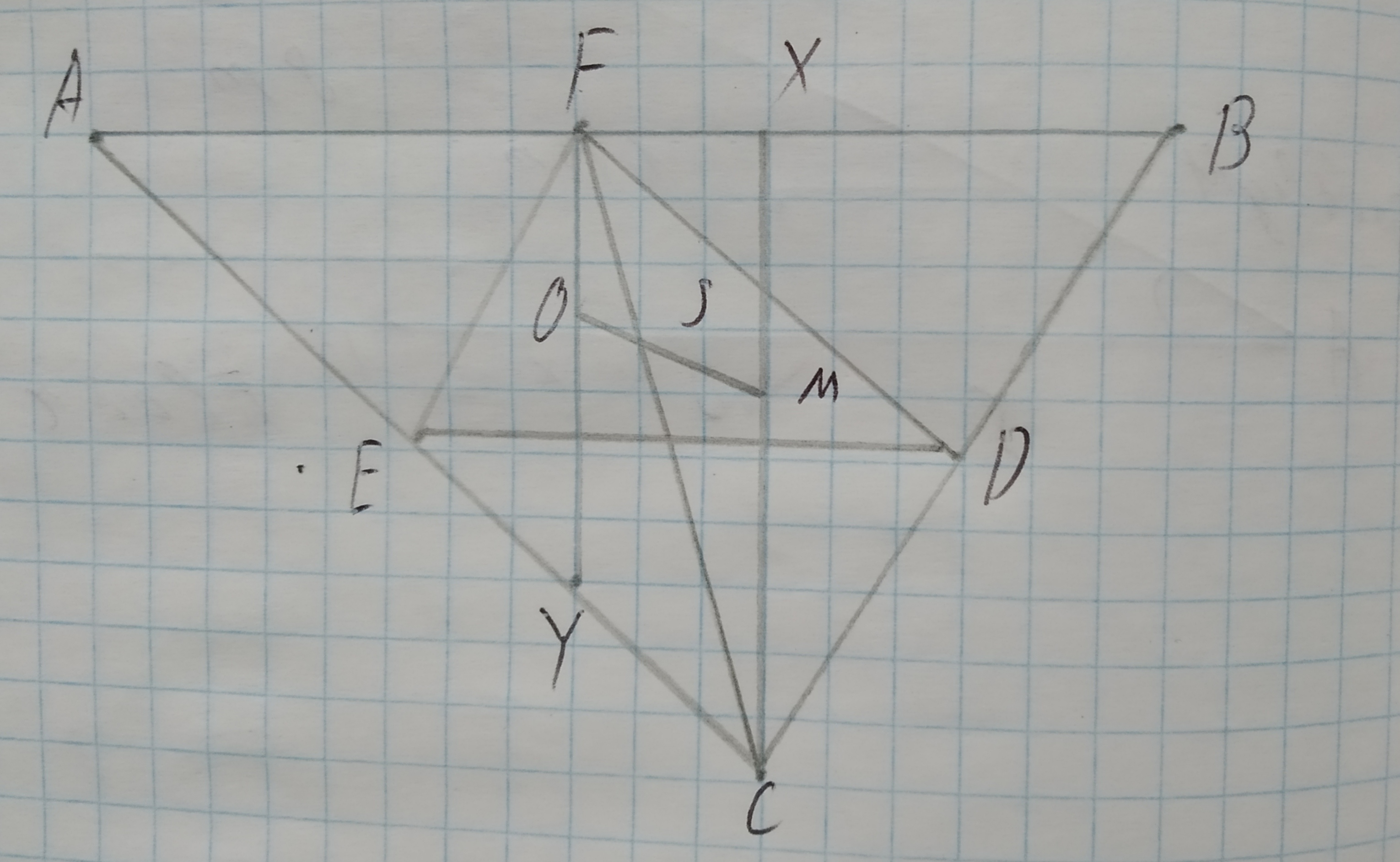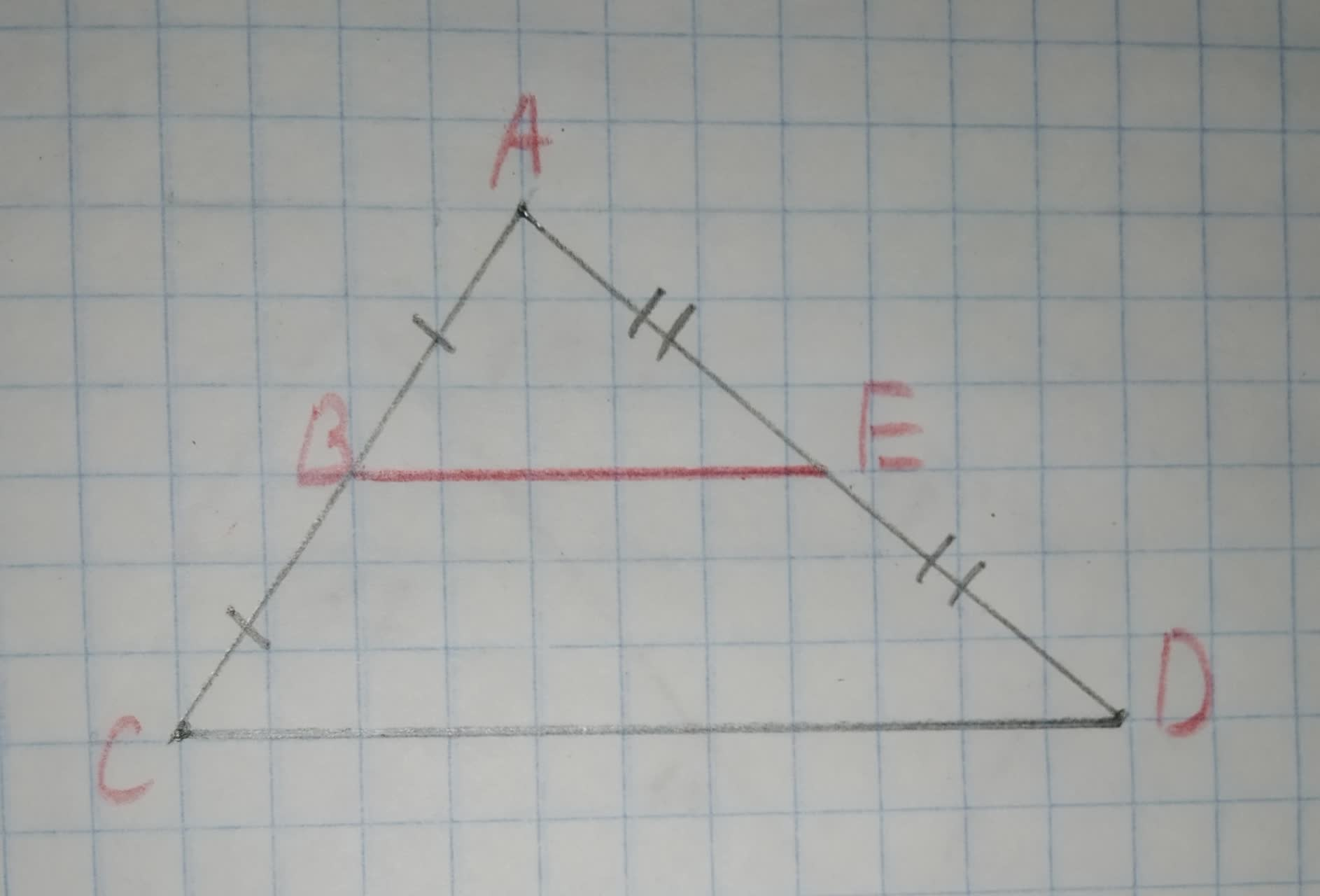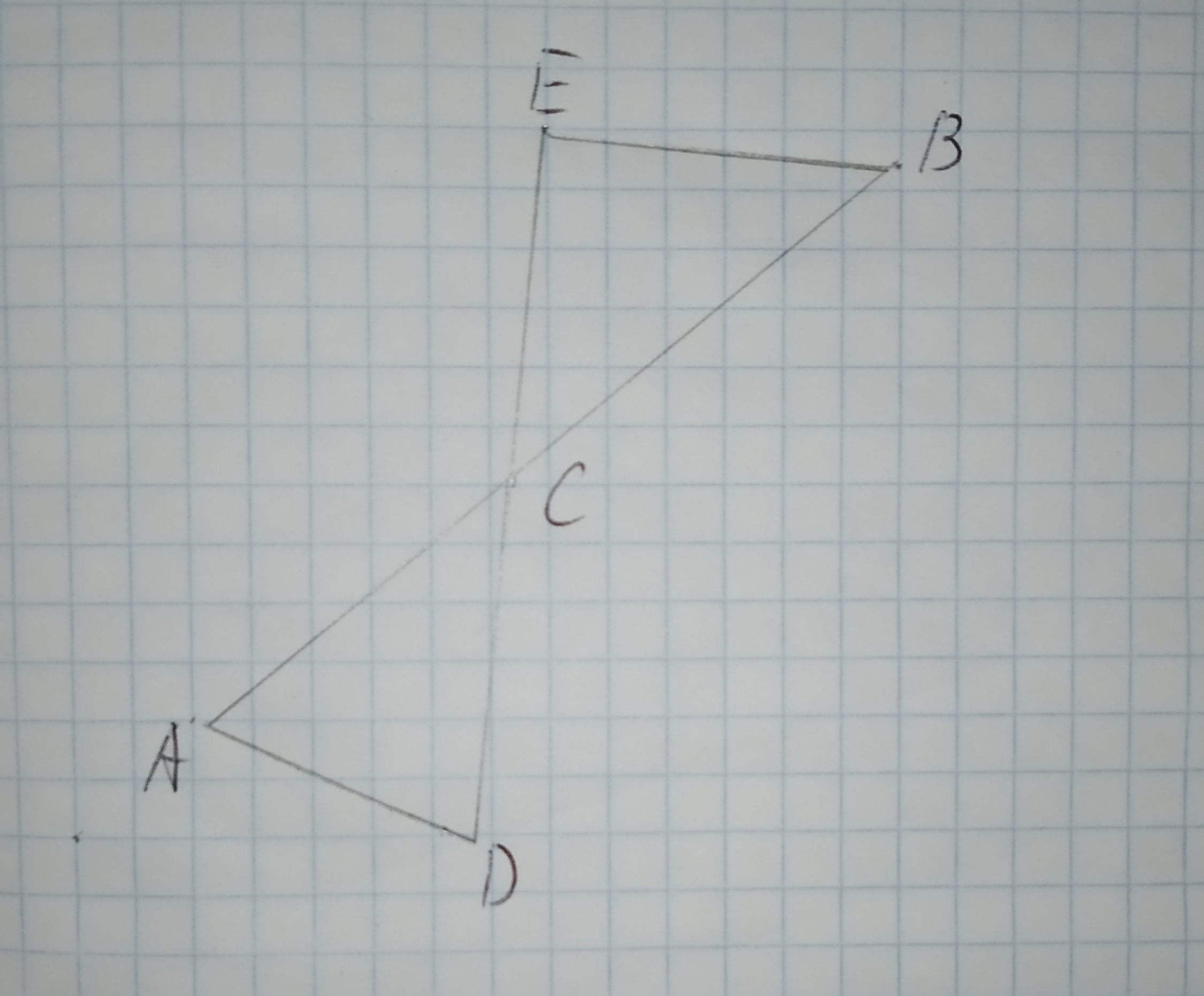# (Euler line) Prove that the orthocenter M, the center O of the circumscribed circle and the barycenter S are collinear. The point S divides the segment OM in the ratio 1:2.Question
Similarity(Euler line) Prove that the orthocenter M, the center O of the circumscribed circle and the barycenter S are collinear. The point S divides the segment OM in the ratio 1:2.2021-01-14
Step 1
We know that, the line on which orthocenter, circumcenter and barycenter(centroid) lie is called Euler Line of the triangle.
Now let a triangle $$\displaystyle\triangle{A}{B}{C}$$ and its medial triangle is $$\displaystyle\triangle{D}{E}{F}$$Here O is orthocenter, M is circumcenter and S is barycenter.
Here we can say $$\displaystyle\triangle{A}{B}{C}$$ is similar to $$\displaystyle\triangle{D}{E}{F}$$ because D, E and F are the midpoint of lines BC, AC and AB respectively.
And also
$$\displaystyle{B}{C}{\mid}{\mid}{E}{F}$$
$$\displaystyle{A}{C}{\mid}{\mid}{F}{D}$$
$$\displaystyle{A}{B}{\mid}{\mid}{E}{D}$$
Hence
$$\displaystyle\triangle{A}{B}{C}\sim\triangle{D}{E}{F}$$ with 2:1 ratio.
In the above figure O is the circumcenter of $$\displaystyle\triangle{A}{B}{C}$$ which is also the orthocenter of $$\displaystyle\triangle{D}{E}{F}$$.
Now we have to prove O, S and M are collinear i.e., O, S and M are at the same line.
Step 2
To prove O, S and M are collinear,
we have to prove
$$\displaystyle\triangle{F}{O}{S}\sim\triangle{C}{M}{S}$$
Since $$\displaystyle{C}{X}\bot{A}{B}$$ and FY is the perpendicular bisector of AB. So we can say
$$\displaystyle{C}{X}{\mid}{\mid}{F}{Y}$$
So alternate interior angle of transversal, when transversal intersect two parallel lines are congruent.
So
$$\displaystyle\angle{S}{F}{O}=\angle{S}{C}{M}$$
also we know that centroid S, splits the median into 2:1 ratio.
that is
CS=2FS
here M is the orthocenter of $$\displaystyle\triangle{A}{B}{C}$$ and O is the orthocenter of $$\displaystyle\triangle{D}{E}{F}$$.
and $$\displaystyle\triangle{A}{B}{C}\sim\triangle{D}{E}{F}$$ with 2:1 ratio.
So we have CM=2FO
Hence we can say
$$\displaystyle\triangle{F}{O}{S}\sim\triangle{C}{M}{S}$$ by SAS similarity.
So we can say
$$\displaystyle\angle{F}{S}{O}=\angle{C}{S}{M}$$
Hence we can say O, S and M are collinear and S divides the line segment MO in the ratio 1:2.

### Relevant QuestionsTo prove:The congruency of $$\displaystyle\vec{{{S}{O}}}\stackrel{\sim}{=}\vec{{{T}{O}}}$$.
Given information: The following information has been given O is the center of the circle
$$\displaystyle\angle{S}{O}{V}=\angle{T}{O}{W}$$
$$\displaystyle\angle{W}{S}{O}=\angle{V}{T}{O}$$Draw a graph for the original figure and its dilated image. Check whether the dilation is a similarity transformation or not.
Given:
The given vertices are
M(1,4), P(2,2), Q(5,5), S(-3,6),T(0,0),U(9,9)Let C be a circle, and let P be a point not on the circle. Prove that the maximum and minimum distances from P to a point X on C occur when the line X P goes through the center of C. [Hint: Choose coordinate systems so that C is defined by
$$x2 + y2 = r2$$ and P is a point (a,0)
on the x-axis with a $$\neq \pm r,$$ use calculus to find the maximum and minimum for the square of the distance. Don’t forget to pay attention to endpoints and places where a derivative might not exist.]Determine whether $$\displaystyle\triangle{A}{B}{C}{\quad\text{and}\quad}\triangle{D}{E}{F}$$ are similar for each set of measures. If so, identify the similarity criterion.
1.$$\displaystyle{m}\angle{A}={20},{m}\angle{C}={20},{m}\angle{D}={40},{m}\angle{F}={40}$$
2.$$\displaystyle{m}\angle{A}={20},{m}\angle{C}={4}{u},{m}\angle{D}={20},{m}\angle{F}={40}$$
3.$$\displaystyle{A}{B}={20},{B}{C}={40},{m}\angle{B}={53}$$,
$$\displaystyle{D}{E}={10},{E}{F}={20},{m}\angle{E}={53}$$To calculate:The ratio of line AB and AC.
Given information:To calculate:The ratio of line AB and BC.
Given information:
The following diagram is givenTo determine: The ratio of the sides of the triangles ABC and GHI.
Given:
Triangle ABC that is 75% of its corresponding side in triangle DEF.
Triangle GHI that is 32% of its corresponding side in triangle DEF.Four point charges are positioned on the rim of a circle. Thecharge on each of the four (in microCoulombs) is +0.5, +1.5, -1.0,and -0.5. If we are told that the electrical potential at thecenter of the circle due to the +0.5 charge is 4.5 x 10^4 V, whatis the potential at the center due to the four chargescombined?To check: whether the additional information in the given option would be enough to prove the given similarity.
Given:
The given similarity is $$\displaystyle\triangle{A}{D}{C}\sim\triangle{B}{E}{C}$$The given options are:
A.$$\displaystyle\angle{D}{A}{C}{\quad\text{and}\quad}\angle{E}{C}{B}$$ are congruent.
B.$$\displaystyle\overline{{{A}{C}}}{\quad\text{and}\quad}\overline{{{B}{C}}}$$ are congruent.
C.$$\displaystyle\overline{{{A}{D}}}{\quad\text{and}\quad}\overline{{{E}{B}}}$$ are parallel.
D.$$\displaystyle\angle{C}{E}{B}$$ is a right triangle.